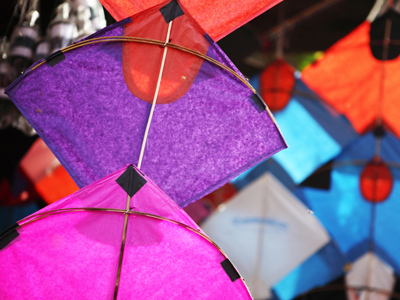A kite shape has two pairs of adjacent sides equal in length.

# Level 3-4 Shapes - Quadrilaterals

As part of your work in KS3 Maths, you'll have come across a variety of shapes. Circles, triangles, pentagons and more. This particular quiz is all about quadrilaterals.

Quadrilaterals are two dimensional shapes that have four sides and four angles. Squares and oblongs spring to mind but there are different kinds of quadrilaterals, each with different properties. As their name suggests, quadrilaterals have four sides (this comes from Latin, with QUAD meaning 4, and LATERAL meaning sides). What other properties do they have? Well, the interior angles of a quadrilateral always add up to 360o.

There are some other rules, such as lengths of sides or whether they are parallel which classify quadrilaterals into different types - rhomboids or trapeziums for example. Try the quiz that follows and see how well you know your quadrilaterals.

1.
What makes an isosceles trapezium different to an 'ordinary' trapezium?
A line of symmetry
No rotational symmetry
Non-parallel lines of equal length
One pair of parallel lines
Just as an isosceles triangle has two sides of equal length, so too with an isosceles trapezium which makes it symmetrical
2.
Which of the following does a parallelogram NOT have?
A line of symmetry
Four angles
Opposite angles are equal
Opposite sides which are equal in length
If it did have a line of symmetry then it would be either a rhombus or a rectangle
3.
How many angles of a square MUST be 90 degrees?
2
3
4
None
All four sides MUST be equal too
4.
The interior angles of a quadrilateral add up to how many degrees?
90
180
360
720
By adding a diagonal you can split a quadrilateral into 2 triangles. The sum of the angles in a triangle is 180o, so the sum of two triangles will be 360o
5.
Which of these shapes is NOT a quadrilateral?
Circle
Rectangle
Rhombus
Square
A circle does not have four sides
6.
How many sides of a trapezium MUST be parallel?
2
3
4
None
The definition of a trapezium is a quadrilateral with at least one pair of parallel sides
7.
Here are four shapes. Which one is NOT a quadrilateral?
Kite
Parallelogram
Trapezium
Triangle
A triangle does not have four sides
8.
A scalene quadrilateral has no sides which are .......
curved
parallel
equal
smooth
Just as a scalene triangle has sides of unequal length, the same is true of a scalene quadrilateral
9.
What is a true statement about a kite?
All four sides are equal in length
Has one pair of parallel sides
Has two pairs of adjacent sides equal in length
It has no lines of symmetry
A kite is a quadrilateral whose four sides can be grouped into two pairs. Each pair is adjacent and of equal-length
10.
How does a rhombus differ from a parallelogram?
All sides are equal in length
Opposite angles are equal
Opposite sides are parallel
Rotational order of symmetry is two
A rhombus is like a 'squashed' square or a diamond